# NCERT SOLUTION FOR CLASS 8 MATHS CHAPTER 7 CUBES AND CUBE ROOTS

The National Council of Educational Research and Training (NCERT) is an autonomous body of the Indian government that formulates the curricula for schools in India that are governed by the Central Board of Secondary Education (CBSE) and certain state boards. Therefore, students who will be taking the Class 10 tests administered by various boards should consult this NCERT Syllabus in order to prepare for those examinations, which in turn will assist those students to get a passing score.

You can also download the NCERT SOLUTION FOR CLASS 8 MATHS CHAPTER 7 CUBES AND CUBE ROOTS to assist you in comprehensively reviewing the course material and achieving a higher score on your upcoming assessments.

Students are able to create a strong conceptual basis with the help of the NCERT Solutions PDFs available at SWC. This base plays a key part in the students’ preparation for the latter phases of the competitive examinations. We give comprehensive answers to challenges posed by NCERT in accordance with the methodology provided by CBSE. The students are able to effortlessly prepare all of the ideas that are presented in their respective courses better and more successfully, and they are even able to succeed in passing the most difficult competitive examinations, such as JEE Main, JEE Advanced, NEET, AIMS, and so on.

Your academic journey begins a new stage as you reach the eighth grade, and a new facet of learning awaits you. This is the year where pupils begin to acquire an interest in the topic at hand, and it is also the year in which you choose whether you will study business, humanity, or science in the following year (class 12). As a consequence of this, having a solid foundation of knowledge in fields such as mathematics and physics comes highly recommended. The NCERT textbook for class 8 is the greatest book now available on the market for enhancing one’s education in the areas of mathematics and science. Read up on the theory that is presented in the book for grade 8 and answer all of the questions that are posed in the practice section of the NCERT textbook.

## NCERT Solutions for Class 8 Maths Chapter 7 – Exercises

### NCERT Solutions for Class 8 Maths Exercise 7.1

Question 1.

Which of the following numbers are not perfect cubes:
(i)216
(ii)128
(iii)1000
(iv)100
(v)46656

Solution :

(i)216
Prime factors of 216 = 2×2×2×3×3×3
Here all factors are in groups of 3’s (in triplets)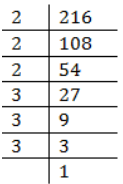Therefore, 216 is a perfect cube number.
(ii) 128
Prime factors of 128
= 2×2×2×2×2×2×2
Here one factor 2 does not appear in a 3’s group.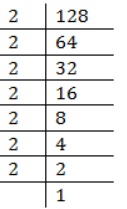Therefore, 128 is not a perfect cube.
(iii) 1000
Prime factors of 1000 = 2×2×2×3×3×3
Here all factors appear in 3’s group.
Therefore, 1000 is a perfect cube.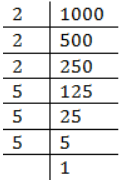(iv) 100
Prime factors of 100 = 2 x 2 x 5 x 5
Here all factors do not appear in 3’s group.
Therefore, 100 is not a perfect cube.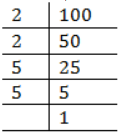(v) 46656
Prime factors of 46656
2x2x2x2x2x2x3x3x3x3x3x3
Here all factors appear in 3’s group.
Therefore, 46656 is a perfect cube.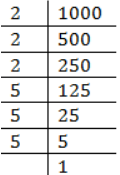Question 2. Find the smallest number by which each of the following numbers must be multiplied to obtain a perfect cube:
(i)243
(ii)256
(iii)72
(iv)675
(v)100

Solution :

(i)243
Prime factors of 243 = 3x3x3x3x3
Here 3 does not appear in 3’s group.
Therefore, 243 must be multiplied by 3 to make it a perfect cube.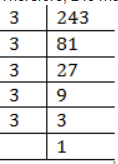(ii) 256
Prime factors of 256
= 2×2×2×2×2×2×2×2
Here one factor 2 is required to make a 3’s group.
Therefore, 256 must be multiplied by 2 to make it a perfect cube.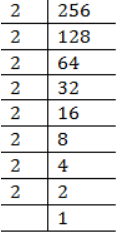(iii) 72
Prime factors of 72 = 2×2×2×3×3
Here 3 does not appear in 3’s group.
Therefore, 72 must be multiplied by 3 to make it a perfect cube.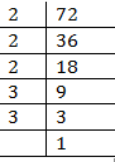(iv) 675
Prime factors of 675 = 3 × 3 × 3 ×  5 × 5
Here factor 5 does not appear in 3’s group.
Therefore 675 must be multiplied by 3 to make it a perfect cube.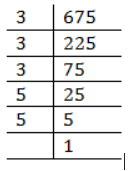(v)  100
Prime factors of 100 =2 × 2 × 5 × 5
Here factor 2 and 5 both do not appear in 3’s group.
Therefore 100 must be multiplied by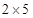= 10 to make it a perfect cube.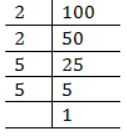Question 3. Find the smallest number by which each of the following numbers must be divided to obtain a perfect cube:
(i)81
(ii)128
(iii)135
(iv)192
(v)704
Solution :

(i)81
Prime factors of 81 = 3 × 3 × 3 × 3
Here one factor 3 is not grouped in triplets.
Therefore 81 must be divided by 3 to make it a perfect cube.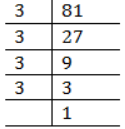(ii) 128
Prime factors of 128 =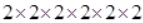Here one factor 2 does not appear in a 3’s group.
Therefore, 128 must be divided by 2 to make it a perfect cube.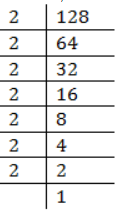(iii) 135
Prime factors of 135 =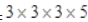Here one factor 5 does not appear in a triplet.
Therefore, 135 must be divided by 5 to make it a perfect cube.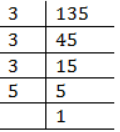(iv) 192
Prime factors of 192 =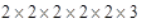Here one factor 3 does not appear in a triplet.
Therefore, 192 must be divided by 3 to make it a perfect cube.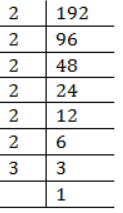(v) 704
Prime factors of 704
=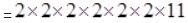Here one factor 11 does not appear in a triplet.
Therefore, 704 must be divided by 11 to make it a perfect cube.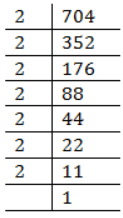Question 4. Parikshit makes a cuboid of plasticine of sides 5 cm, 2 cm, 5 cm. How many such cuboids will he need to form a cube?
Ans. Given numbers =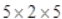Since, Factors of 5 and 2 both are not in group of three.
Therefore, the number must be multiplied by 2 × 5 × 2 = 20 to make it a perfect cube.
Hence he needs 20 cuboids.

### NCERT Solutions for Class 8 Maths Exercise 7.2

Question 1.

Find the cube root of each of the following numbers by prime factorization method:
(i) 64
(ii) 512
(iii) 10648
(iv) 27000
(v) 15625
(vi) 13824
(vii) 110592
(viii) 46656
(ix) 175616
(x) 91125

Solution : (i) 64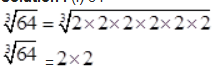= 4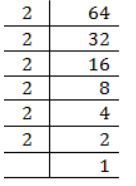(ii) 512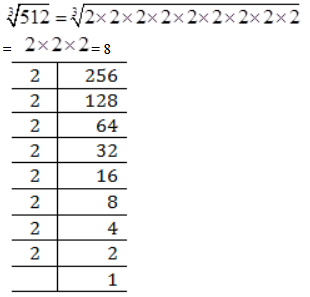(iii) 10648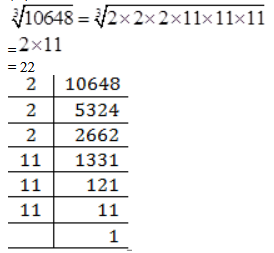(iv) 27000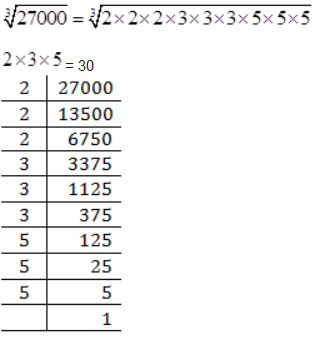(v) 15625

=5 × 5
= 25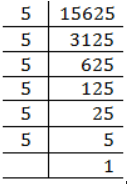(vi) 13824

=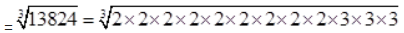= 24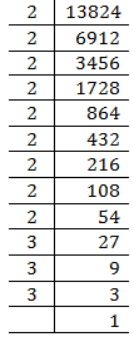(vii) 110592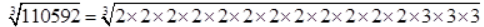2×2×2×2×3
= 48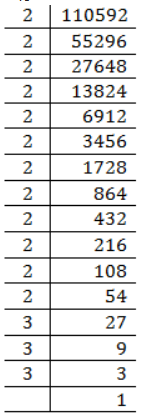(viii) 46656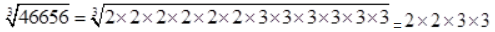= 36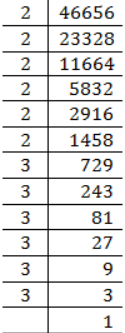(ix) 175616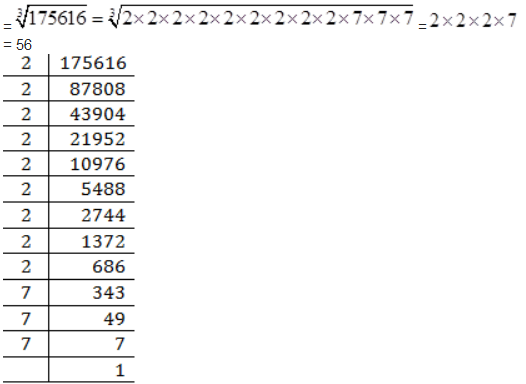= 56

(x) 91125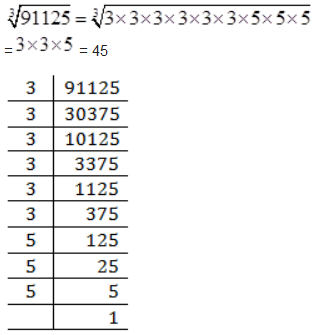Question 2.

State true or false:
(i) Cube of any odd number is even.
(ii) A perfect cube does not end with two zeroes.
(iii) If square of a number ends with 5, then its cube ends with 25.
(iv) There is no perfect cube which ends with 8.
(v) The cube of a two digit number may be a three digit number.
(vi) The cube of a two digit number may have seven or more digits.
(vii) The cube of a single digit number may be a single digit number.

Solution : (i) False
Since,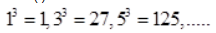are all odd.
(ii) True
Since, a perfect cube ends with three zeroes. e.g.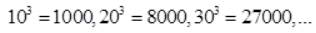so on

(iii) False
Since,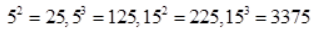(Did not end with 25)

(iv) False
Since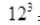= 1728
[Ends with 8]
And  = 10648
[Ends with 8]

(v) False Since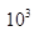= 1000
[Four digit number]
And  11³ = 1331
[Four digit number]
(vi) False Since  99³ = 970299
[Six digit number]

(vii) True
= 1
[Single digit number]
2³ = 8
[Single digit number]

Question 3.

You are told that 1,331 is a perfect cube. Can you guess with factorization what is its cube root? Similarly guess the cube roots of 4913, 12167, 32768.
Solution :

We know that 10³ = 1000 and Possible cube of  11³ = 1331
Since, cube of unit’s digit = 1
Therefore, cube root of 1331 is 11.
4913
We know that  7³ = 343
Next number comes with 7 as unit place  17³ = 4913
Hence, cube root of 4913 is 17.
12167
We know that = 27
Here in cube, ones digit is 7
Now next number with 3 as ones digit
13³ = 2197
And next number with 3 as ones digit
23³ = 12167
Hence cube root of 12167 is 23.
32768
We know that  2³ = 8
Here in cube, ones digit is 8
Now next number with 2 as ones digit
12³ = 1728
And next number with 2 as ones digit
22³ = 10648
And next number with 2 as ones digit
32³ = 32768
Hence cube root of 32768 is 32.

## Conclusions for NCERT Solutions Class 8 Maths Chapter 7

The academic team of SWC has provided NCERT answers for class 8 mathematics chapter-7. We’ve given solutions for all of Chapter 7’s exercises. These are step-by-step answers to all of the problems in Chapter 7 of the NCERT textbook. Read the first theoretical chapter. Make sure you’ve gone through the theoretical section of chapter 7 in the NCERT textbook and that you know the formula for the chapter.

When working through the exercises in the NCERT textbook, if you run into any type of difficulty or uncertainty, you may use the SWC NCERT Solutions for class 8 as a point of reference. While you are reading the theory from the textbook, it is imperative that you always have notes prepared. You should make an effort to understand things from the very beginning so that you may create a solid foundation in the topic. Use the NCERT as your parent book to ensure that you have a strong foundation. After you have finished reading the theoretical section of the textbook, you should go to additional reference books.